Published

# Christmas Gift Box

Christmas Gift Box plays music and sends an email when it is opened.

IntermediateFull instructions provided5,120## Things used in this project

### Hardware components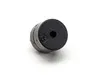Buzzer
×3Particle Photon
×3
 Magnetic Sensor Switch for Arduino
×3×3
 Led Matrix Driver Module
×3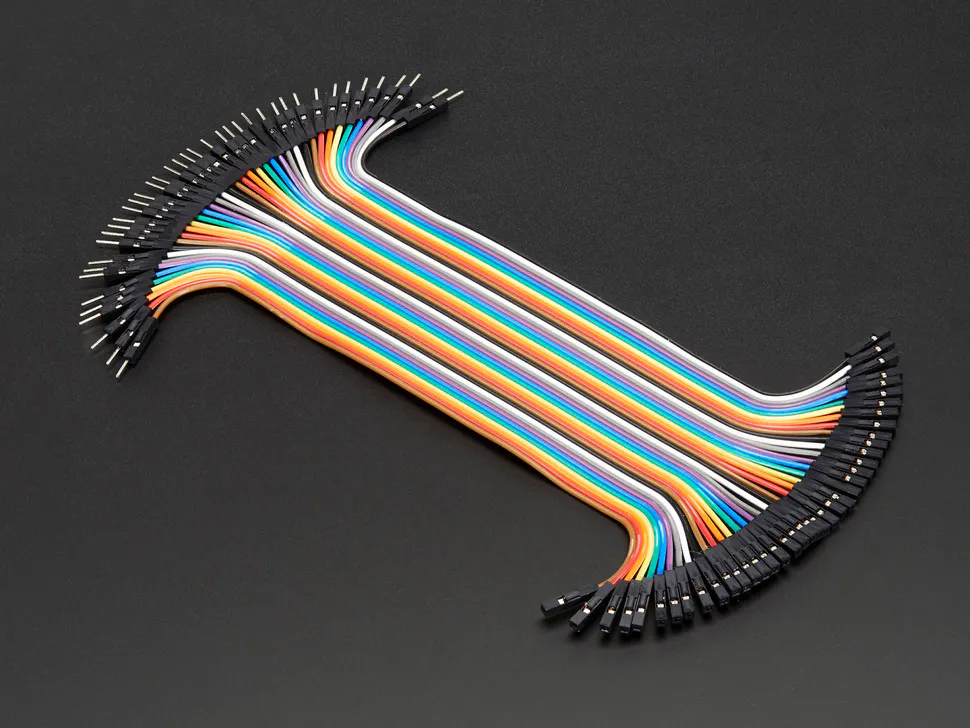Male/Female Jumper Wires
×1

### Software apps and online services

 Fritzing
 ParticleUbidots

## Schematics

### Flow Diagram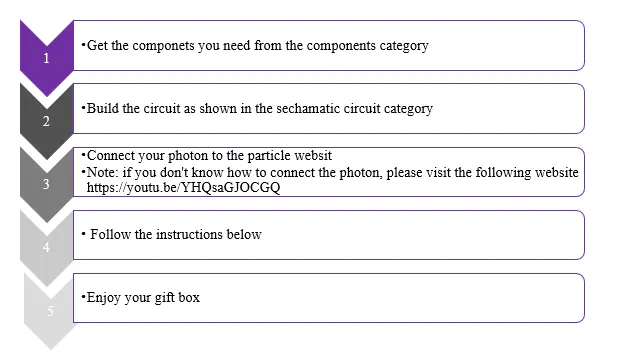### Circuit Connections

The following tables illustrate the connections.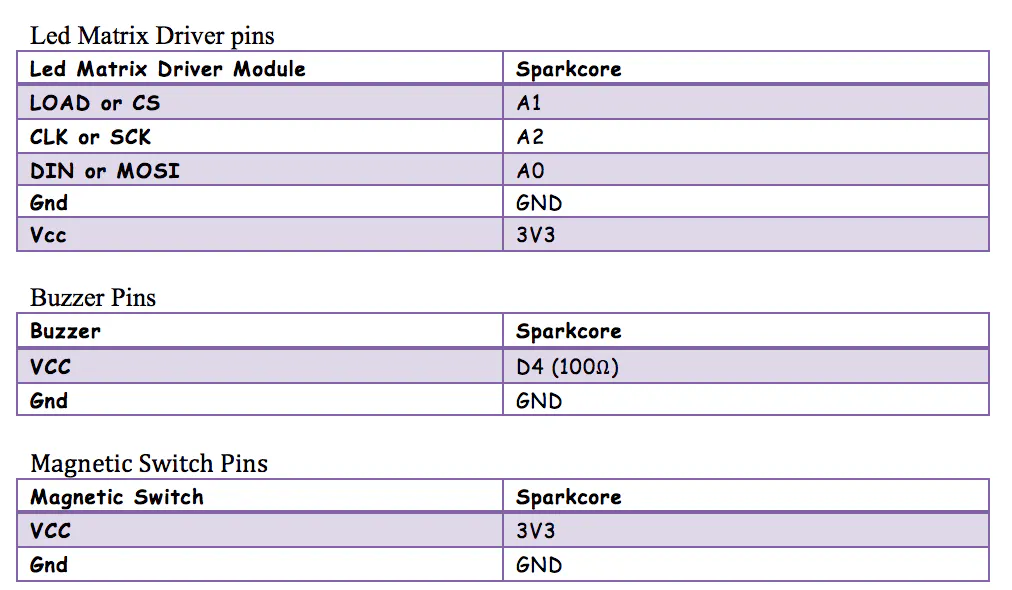### Circuit Diagram

A simple simulation of the project circuit.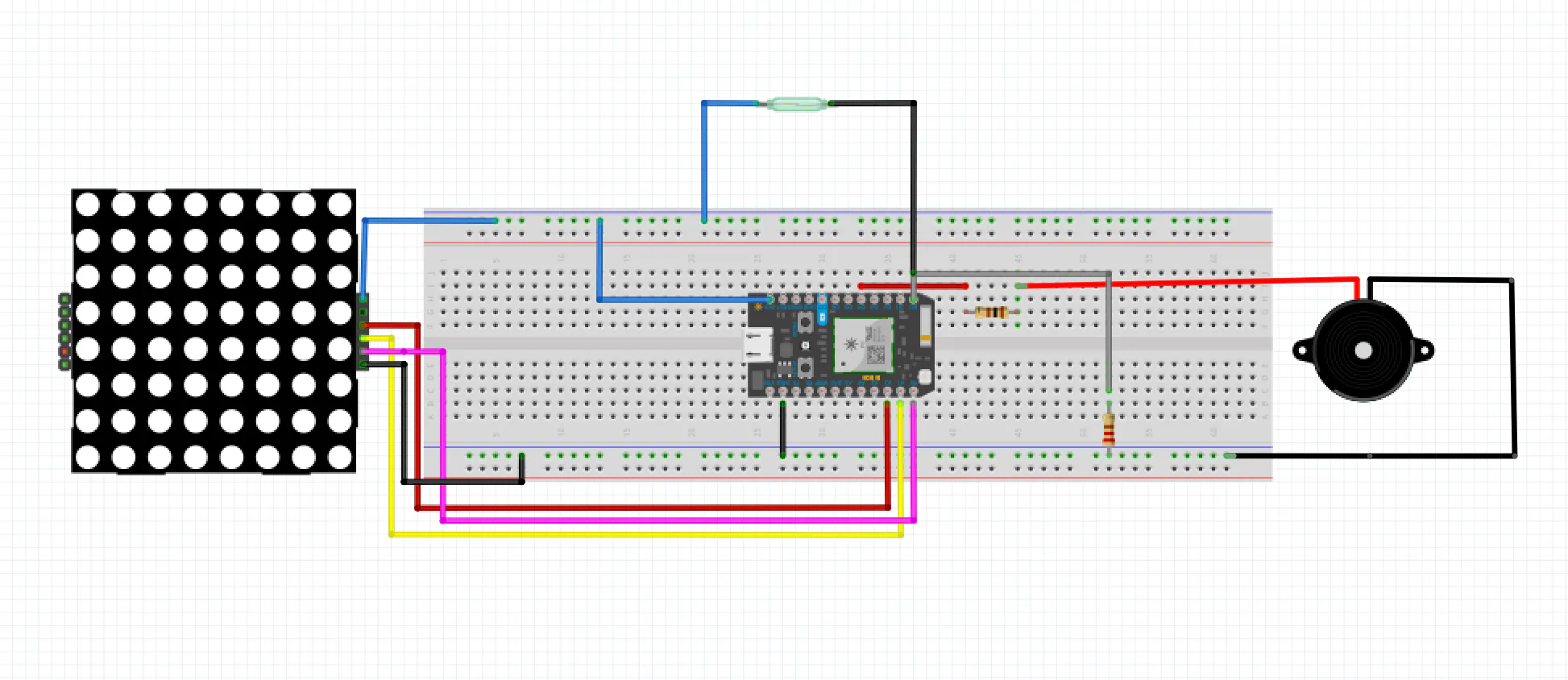### Circuit Wiring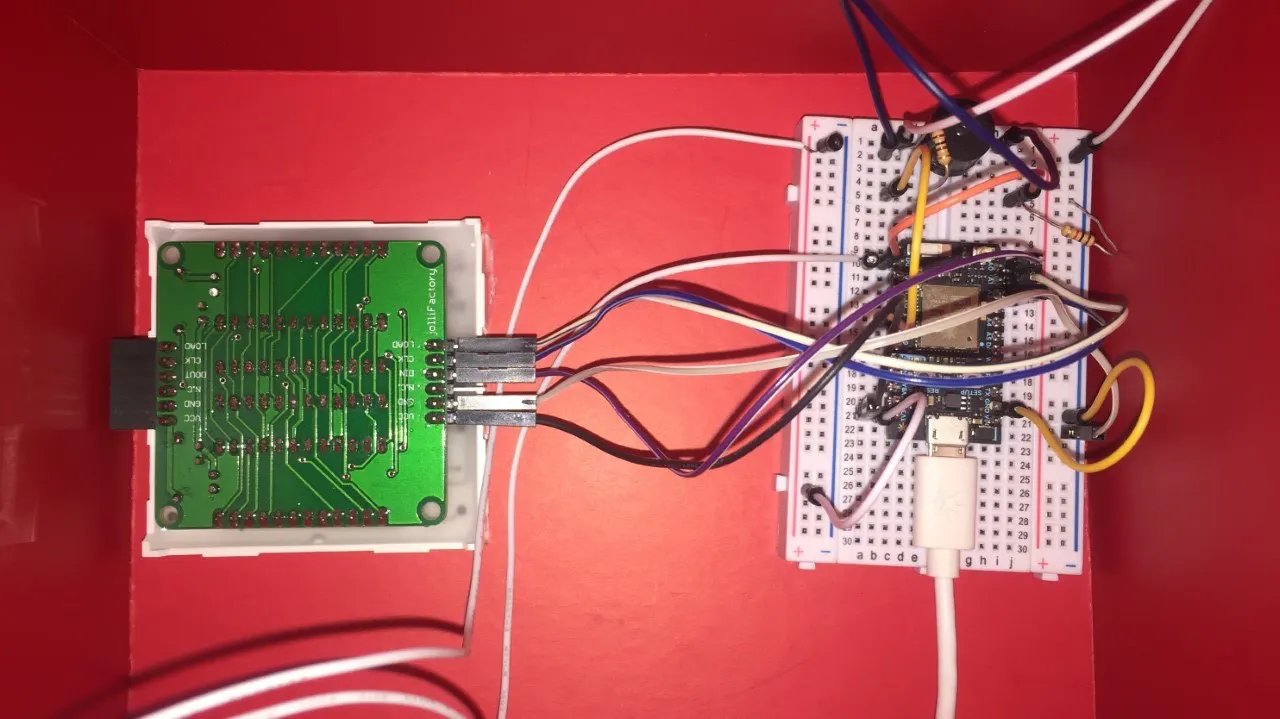### Dashboard Setup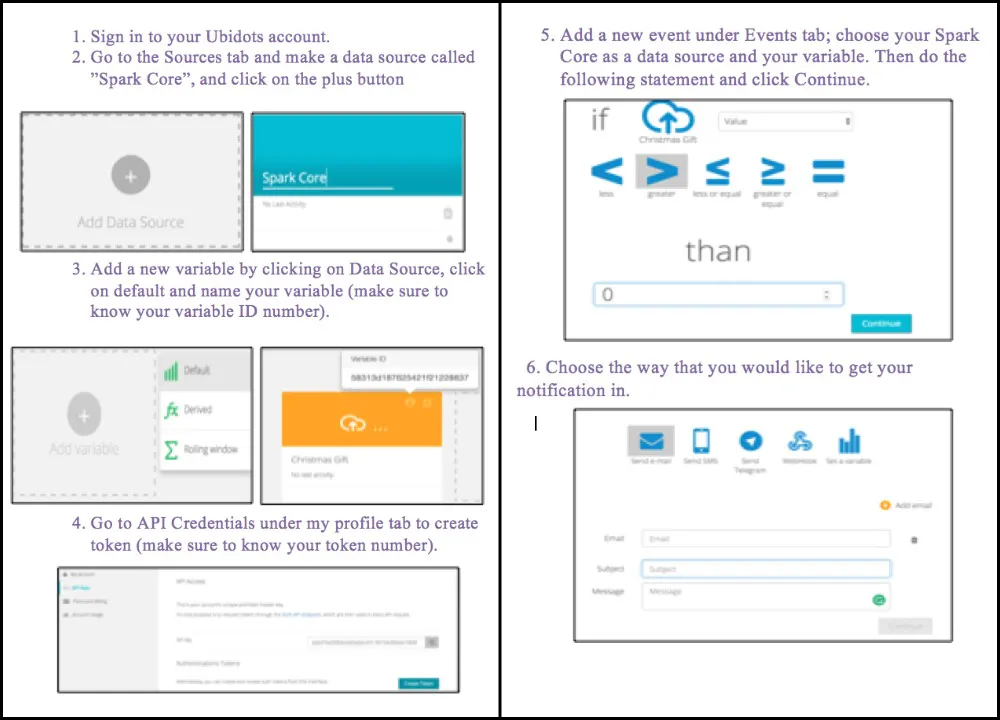### Graphs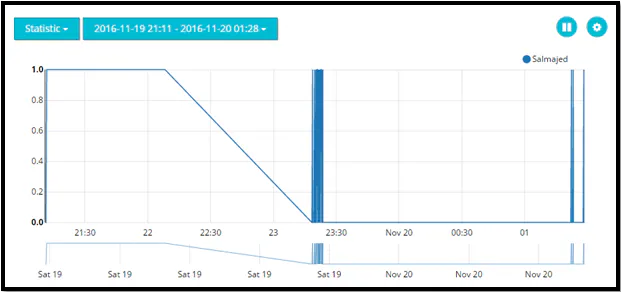### Data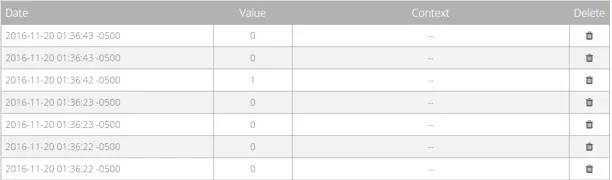### LED Matrix Driver Components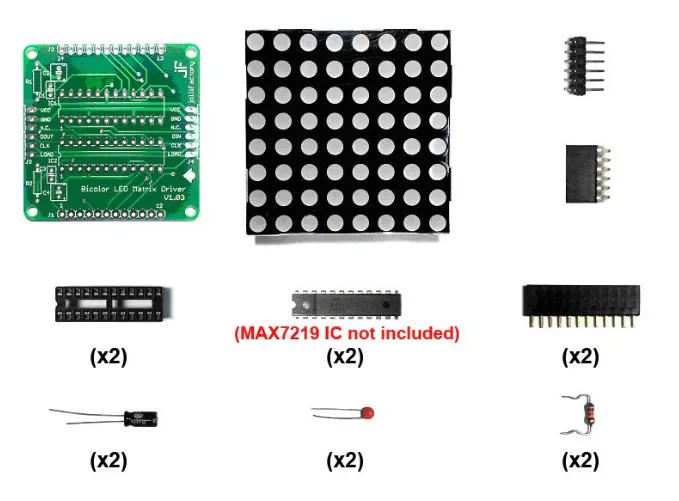## Code

### The Project Code for One Particle

C/C++
```// This #include statement was automatically added by the Particle IDE.
#include "LedControl-MAX7219-MAX7221/LedControl-MAX7219-MAX7221.h"

// This #include statement was automatically added by the Particle IDE.
#include "HttpClient/HttpClient.h"

#define VARIABLE_ID "5830e82c76254232aaee303b" //Change here your variable ID and token
#define TOKEN "ZD8DmKxKIcCT520EM8YOzLJNHyYBN5"

int open=0;
LedControl *led;// LedControl object
HttpClient http;
uint8_t data = A0;
uint8_t load = A1;
uint8_t myclock = A2;

int noteFreqArr[] = {
49.4, 52.3, 55.4, 58.7, 62.2, 65.9, 69.9, 74, 78.4, 83.1, 88, 93.2,
98.8, 105, 111, 117, 124, 132, 140, 148, 157, 166, 176, 186,
198, 209, 222, 235, 249, 264, 279, 296, 314, 332, 352, 373,
395, 419, 444, 470, 498, 527, 559, 592, 627, 665, 704, 746,
790, 837, 887, 940, 996, 1050, 1110, 1180, 1250, 1320, 1400, 1490,
1580, 1670, 1770, 1870, 1990, 2100,2210, 2340, 2480,2630,2790 };

{ "Content-Type", "application/json" },
{ "X-Auth-Token" , TOKEN },
{ NULL, NULL } // NOTE: Always terminate headers will NULL
};

http_request_t request;
http_response_t response;

void setup() {
pinMode(D0, INPUT);
request.hostname = "things.ubidots.com";
request.port = 80;
request.path = "/api/v1.6/variables/"VARIABLE_ID"/values";
pinMode(D4, OUTPUT);
Serial.begin(9600);
led = new LedControl(data,myclock,load,1); //DIN,CLK,CS,HowManyDisplays
led-> shutdown(0,false); //Turn it on
led-> setIntensity(0,7);//Set Led's Intensity, max value=15
}

void loop() {
//Serial.print("open: ");
//Serial.println(open);
if (open==0){
init();
open=1;
}
else{
open=0;
}
request.body = "{\"value\":" + String(open) + "}"; //Sending 1 if the box is open to Ubidots
}

// The next 2 functions were written by Rob Faludi and Christopher Stevens.

void playNote(int noteInt, long length) //first parameter is the index of the frequency vector and the second is the duration of the note in ms
{
long breath = 20;
length = length - breath;
buzz(4, noteFreqArr[noteInt], length);
if(breath > 0) { //take a short pause or 'breath' if specified
delay(breath);
}

}

void buzz(int targetPin, long frequency, long length)
{
long delayValue = 1000000/frequency/2; // calculate the delay value between transitions
//// 1 second's worth of microseconds, divided by the frequency, then split in half since
//// there are two phases to each cycle
long numCycles = frequency * length/ 1000; // calculate the number of cycles for proper timing
//// multiply frequency, which is really cycles per second, by the number of seconds to
//// get the total number of cycles to produce
for (long i=0; i < numCycles; i++){ // for the calculated length of time...
digitalWrite(D4,HIGH); // write the buzzer pin high to push out the diaphram
delayMicroseconds(delayValue); // wait for the calculated delay value
digitalWrite(D4,LOW); // write the buzzer pin low to pull back the diaphram
delayMicroseconds(delayValue); // wait againf or the calculated delay value
}
}

void putByte(byte data_1)
{
byte i = 8;
while(i > 0)
{
mask = 0x01 << (i - 1); // get bitmask

digitalWrite(myclock, LOW); // tick

if (data_1 & mask) // choose bit
{
digitalWrite(data, HIGH);// send 1
}
else
{
digitalWrite(data, LOW); // send 0
}
digitalWrite(myclock, HIGH); // tock
--i; // move to lesser bit
}
}

void selectColor(int cmd)
{
byte reg = 0x0c;  //max7219_reg_shutdown
byte col = 0x01;  //shutdown false
byte col2 = 0x00;  //shutdown true
int c = 0;
if (cmd == 0)//Off
{
for ( c =1; c<= 4; c++) {
putByte(reg);// specify register
putByte(col2);//((data & 0x01) * 256) + data >> 1); // put data

putByte(reg);// specify register
putByte(col2);//((data & 0x01) * 256) + data >> 1); // put data
}
}
else if (cmd == 2)//Green
{
led-> setIntensity(0,14);

for ( c =1; c<= 4; c++) {
putByte(reg);// specify register
putByte(col);//((data & 0x01) * 256) + data >> 1); // put data

putByte(reg);// specify register
putByte(col2);//((data & 0x01) * 256) + data >> 1); // put data
}
}
else if (cmd == 3)//Red
{

led-> setIntensity(0,3);
for ( c =1; c<= 4; c++)
{
putByte(reg);// specify register
putByte(col2);//((data & 0x01) * 256) + data >> 1); // put data

putByte(reg);// specify register
putByte(col);//((data & 0x01) * 256) + data >> 1); // put data
}
}
}

void matrix(int color){
selectColor(color);
for(int i=0; i<8; i++){
led->setColumn(0,i,0xFF);
}
}
//A little bit of the song 12 days of christmas
void init(void){
matrix(2);
playNote(44,291);
playNote(44,291);
playNote(44,582);
matrix(3);
playNote(49,291);
playNote(49,291);
playNote(49,582);
playNote(48,291);
playNote(49,291);
matrix(2);
playNote(51,291);
playNote(53,291);
playNote(54,291);
playNote(51,291);
matrix(3);
playNote(53,582);
delay(291);
playNote(54,291);
playNote(56,582);
playNote(58,291);
playNote(54,291);
matrix(2);
playNote(53,291);
playNote(49,291);
playNote(51,582);
matrix(3);
playNote(49,1164);
matrix(2);
//More Notes if you want a larger song
// playNote(56,291);
//playNote(56,291);
//playNote(56,582);
//playNote(61,291);
//matrix(3);
//playNote(61,291);
//playNote(61,582);
//playNote(60,291);
//playNote(61,291);
//matrix(2);
//playNote(63,291);
//playNote(65,291);
//playNote(66,291);
//playNote(63,291);
//matrix(3);
//playNote(65,1164);
//matrix(2);
//playNote(68,582);
//playNote(51,291);
//playNote(53,291);
//playNote(54,582);
// matrix(3);
//playNote(65,291);
//playNote(66,291);
//playNote(68,582);
//playNote(71,291);
//matrix(2);
//playNote(66,291);
//playNote(65,291);
//playNote(61,291);
//playNote(63,582);
// matrix(3);
//playNote(61,1445);
matrix(2);
playNote(48,291);
playNote(48,291);
playNote(48,582);
matrix(3);
playNote(53,291);
playNote(53,291);
playNote(53,582);
matrix(0);
}
```

### The Project Code for more than One Particle

C/C++
```#include "LedControl-MAX7219-MAX7221/LedControl-MAX7219-MAX7221.h"

// This #include statement was automatically added by the Spark IDE.
#include "HttpClient/HttpClient.h"

#define VARIABLE_ID "5830e80f76254231fafe8f13" //Change here your variable ID and token
#define TOKEN "farSf05NfW3hNXUqbFjWi2RtWRrUhi"

#define VARIABLE_ID2 "582bd71076254256d39c09bc" // device 2
#define VARIABLE_ID3 "5830e82c76254232aaee303b" // device 3

int open=0;
LedControl *led;// LedControl object
HttpClient http;
uint8_t data = A0;
uint8_t load = A1;
uint8_t myclock = A2;

int noteFreqArr[] = {
49.4, 52.3, 55.4, 58.7, 62.2, 65.9, 69.9, 74, 78.4, 83.1, 88, 93.2,
98.8, 105, 111, 117, 124, 132, 140, 148, 157, 166, 176, 186,
198, 209, 222, 235, 249, 264, 279, 296, 314, 332, 352, 373,
395, 419, 444, 470, 498, 527, 559, 592, 627, 665, 704, 746,
790, 837, 887, 940, 996, 1050, 1110, 1180, 1250, 1320, 1400, 1490,
1580, 1670, 1770, 1870, 1990, 2100,2210, 2340, 2480,2630,2790 };

{ "Content-Type", "application/json" },
{ "X-Auth-Token" , TOKEN },
{ NULL, NULL } // NOTE: Always terminate headers will NULL
};

http_request_t request;
http_response_t response;

void setup() {

pinMode(D0, INPUT);
Particle.subscribe("christmasbox", myHandler, "20003c000147353138383138");
request.hostname = "things.ubidots.com";
request.port = 80;
request.path = "/api/v1.6/variables/"VARIABLE_ID"/values";
pinMode(D4, OUTPUT);
Serial.begin(9600);
led = new LedControl(data,myclock,load,1); //DIN,CLK,CS,HowManyDisplays
led-> shutdown(0,false); //Turn it on
led-> setIntensity(0,7);//Set Led's Intensity, max value=15

}

void loop() {
//Serial.print("open: ");
//Serial.println(open);
if (open==0){
init();
open=1;
Particle.publish("christmasbox", open);
delay(3000);

}
else{
open=0;
Particle.publish("christmasbox", open);
delay(3000);

}
request.body = "{\"value\":" + String(open) + "}"; //Sending 1 if the box is open to Ubidots

}

void myHandler(const char *event, const char *data)
{
//this runs when you receive a publish event
if (open==1) {
// if your buddy's beam is intact, then turn your board LED off
}
else if (open==0) {
// if your buddy's beam is broken, turn your board LED on
}
else {
// if the data is something else, don't do anything.
// Really the data shouldn't be anything but those two listed above.
}
}

// The next 2 functions were written by Rob Faludi and Christopher Stevens.

void playNote(int noteInt, long length) //first parameter is the index of the frequency vector and the second is the duration of the note in ms
{
long breath = 20;
length = length - breath;
buzz(4, noteFreqArr[noteInt], length);
if(breath > 0) { //take a short pause or 'breath' if specified
delay(breath);
}

}

void buzz(int targetPin, long frequency, long length)
{
long delayValue = 1000000/frequency/2; // calculate the delay value between transitions
//// 1 second's worth of microseconds, divided by the frequency, then split in half since
//// there are two phases to each cycle
long numCycles = frequency * length/ 1000; // calculate the number of cycles for proper timing
//// multiply frequency, which is really cycles per second, by the number of seconds to
//// get the total number of cycles to produce
for (long i=0; i < numCycles; i++){ // for the calculated length of time...
digitalWrite(D4,HIGH); // write the buzzer pin high to push out the diaphram
delayMicroseconds(delayValue); // wait for the calculated delay value
digitalWrite(D4,LOW); // write the buzzer pin low to pull back the diaphram
delayMicroseconds(delayValue); // wait againf or the calculated delay value
}
}

void putByte(byte data_1)
{
byte i = 8;
while(i > 0)
{
mask = 0x01 << (i - 1); // get bitmask

digitalWrite(myclock, LOW); // tick

if (data_1 & mask) // choose bit
{
digitalWrite(data, HIGH);// send 1
}
else
{
digitalWrite(data, LOW); // send 0
}
digitalWrite(myclock, HIGH); // tock
--i; // move to lesser bit
}
}

void selectColor(int cmd)
{
byte reg = 0x0c;  //max7219_reg_shutdown
byte col = 0x01;  //shutdown false
byte col2 = 0x00;  //shutdown true
int c = 0;
if (cmd == 0)//Off
{
for ( c =1; c<= 4; c++) {
putByte(reg);// specify register
putByte(col2);//((data & 0x01) * 256) + data >> 1); // put data

putByte(reg);// specify register
putByte(col2);//((data & 0x01) * 256) + data >> 1); // put data
}
}
else if (cmd == 2)//Green
{
led-> setIntensity(0,14);

for ( c =1; c<= 4; c++) {
putByte(reg);// specify register
putByte(col);//((data & 0x01) * 256) + data >> 1); // put data

putByte(reg);// specify register
putByte(col2);//((data & 0x01) * 256) + data >> 1); // put data
}
}
else if (cmd == 3)//Red
{

led-> setIntensity(0,3);
for ( c =1; c<= 4; c++)
{
putByte(reg);// specify register
putByte(col2);//((data & 0x01) * 256) + data >> 1); // put data

putByte(reg);// specify register
putByte(col);//((data & 0x01) * 256) + data >> 1); // put data
}
}
}

void matrix(int color){
selectColor(color);
for(int i=0; i<8; i++){
led->setColumn(0,i,0xFF);
}
}
//A little bit of the song 12 days of christmas
void init(void){
matrix(2);
playNote(44,291);
playNote(44,291);
playNote(44,582);
matrix(3);
playNote(49,291);
playNote(49,291);
playNote(49,582);
playNote(48,291);
playNote(49,291);
matrix(2);
playNote(51,291);
playNote(53,291);
playNote(54,291);
playNote(51,291);
matrix(3);
playNote(53,582);
delay(291);
playNote(54,291);
playNote(56,582);
playNote(58,291);
playNote(54,291);
matrix(2);
playNote(53,291);
playNote(49,291);
playNote(51,582);
matrix(3);
playNote(49,1164);
matrix(2);
//More Notes if you want a larger song
playNote(56,291);
playNote(56,291);
playNote(56,582);
playNote(61,291);
matrix(3);
playNote(61,291);
playNote(61,582);
playNote(60,291);
playNote(61,291);
matrix(2);
playNote(63,291);
playNote(65,291);
playNote(66,291);
playNote(63,291);
matrix(3);
playNote(65,1164);
matrix(2);
playNote(68,582);
playNote(51,291);
playNote(53,291);
//playNote(54,582);
// matrix(3);
//playNote(65,291);
//playNote(66,291);
//playNote(68,582);
//playNote(71,291);
//matrix(2);
//playNote(66,291);
//playNote(65,291);
//playNote(61,291);
//playNote(63,582);
// matrix(3);
//playNote(61,1445);
matrix(2);
playNote(48,291);
playNote(48,291);
playNote(48,582);
matrix(3);
playNote(53,291);
playNote(53,291);
playNote(53,582);
matrix(0);
}
```

## Credits

### Rawan Alabbas

1 project • 8 followers

### Shaima Almajed

1 project • 2 followers

### Maram Alzayer

1 project • 5 followers